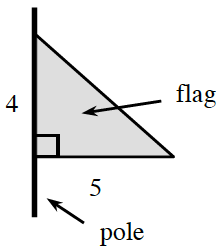### Home > APCALC > Chapter 1 > Lesson 1.2.2 > Problem1-42

1-42.

Imagine rotating the flag at right about its pole.

1. Describe the resulting three-dimensional figure. Draw a picture of this figure on your paper.

To help you visualize the 3D figure, refer back to the hint in problem 1-23.

2. Calculate the volume of the rotated flag.

Prism: $V=Bh$;   Cylinder: $V=\pi r^2h$;   Sphere: $V=\frac{4}{3}πr^3$;  Pyramid: $V=\frac{1}{3}Bh$;  Cone: $V=\frac{1}{3}πr^2h$Use the eTool below to visualize the flag.
Click the link at right for the full version of the eTool: Calc 1-42 HW eTool